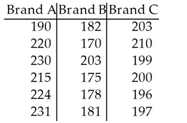The question is...

3) The table below shows the lifetimes (in hours) of random samples of light bulbs of three different brands. Use a 0.01 significance level to test the claim that the different brands have the same median lifetime. Find the critical value for the test:A) Critical value: X2 = 5.991.
B) Critical value: X2 = 4.605.
C) Critical value: X2 = 6.251.
D) Critical value: X2 = 9.210.
E) Critical value: X2 = 11.345.